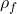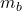## A Submerged Ball Part A A ball of mass mb and volume V is lowered on a string into a fluid of density Pi (Figure 1) Assume that the object w

Question

A Submerged Ball Part A A ball of mass mb and volume V is lowered on a string into a fluid of density Pi (Figure 1) Assume that the object would sink to the bottom if it were not supported by the string. What is the tension T in the string when the ball is fully submerged but not touching the bottom as shown in the figure? Express your answer in terms of any or all of the given quantities and g, the magnitude of the acceleration due to gravity

Note:
a ball is suspended in a fluid

in progress 0
2 months 2021-07-30T03:04:23+00:00 1 Answers 4 views 0

T = mg –×V×g

Explanation:

Here we have the mass of the ball is given asThe density of the fluid =The volume of the ball = V

Acceleration due to gravity = g

According to Archimedes’s principle, the upthrust = Weight of fluid displaced

Tension in string = T = weight of ball – upthrust

T = mg –×V×g